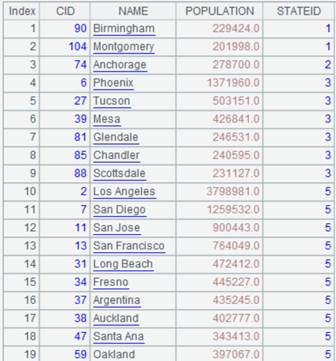• 集算器
教程
函数参考
习题
代码参考
用户参考
外部库使用指南
数据文件工具用户参考
DQL教程
集群管理器使用说明
SPL命令执行器用户参考
• 润乾报表
教程
填报教程
分析教程
报表中心教程
用户参考
程序员参考
高级指南
通用查询
DQL部署集成手册
• 易明建模
用户参考
json参数说明
• 润乾官网

# rank()

## A.rank(y)

A.rank(y)

 @z 从大到小排名。注意：这里是小写的字母"z" @i 先去掉A中的重复成员，然后计算y在其中的排名 @s 先对有重复成员的排名做均值计算，然后返回计算后的排名，可能不是整数。例如：[3,2,6,6,9],常用的排名结果为[2,1,3,3,5]，使用@s选项时，采用平均排名就会变为[2.0,1.0,(3+4)/2,(3+4)/2,5.0]

 A 序列 y A中的成员

 A 1 =[2,1,3,4,8,5,2,0] 2 =A1.rank(6) 8,从小到大的排名 3 =A1.rank@z(6) 2,从大到小的排名 4 =A1.rank@i(2) 3,先去掉重复成员，然后计算排名 5 =A1.rank@s(2) 3.5,先对有重复成员的排名做均值运算，再返回排名

A.rank(y,x)

## A.rank( y,x )

 A.rank(y,x) 相当于A.(x).rank(y)

 @z 从大到小排名。注意：这里是小写的字母“z” @i 先去掉A.(x)中的重复成员，然后计y在其中的排名 @s 先对有重复成员的排名做均值计算，然后返回计算后的排名，可能不是整数。例如：[3,2,6,6,9],常用的排名结果为[2,1,3,3,5]，使用@s选项时，采用平均排名就会变为[2.0,1.0,(3+4)/2,(3+4)/2,5.0]

 x A的计算表达式 y A中的成员或者用于比较A.(x)的值 A 序列

y成员排名

 A 1 =demo.query("select * from SCORES where SUBJECT='English'") 2 =A1.rank(90,SCORE) 23,从小到大排名 3 =A1.rank@z(90,SCORE) 5,从大到小排名 4 =A1.rank@i(90,SCORE) 10,去除重复的成绩后的排名 5 =A1.(SCORE).rank(90) 23 6 =A1.rank@s(90,SCORE) 23.5,先对有重复成员的排名做均值运算，再返回排名

A.rank(y)

## rank()

rank(F;Gi,…)

 F 字段名 Gi 字段名

 A 1 =demo.query("select  SUBJECT,STUDENTID,SCORE from SCORES where CLASS='Class one' order by SUBJECT,SCORE desc") 查询班级为Class one的学生成绩，根据SUBJECT升序同时根据SCORE降序排序 2 =A1.derive(rank(SCORE;SUBJECT): RANKING) 获取单科成绩排名次序，分数相同则名次相同，并列成绩占用名次，结果值保存在RANKING字段中